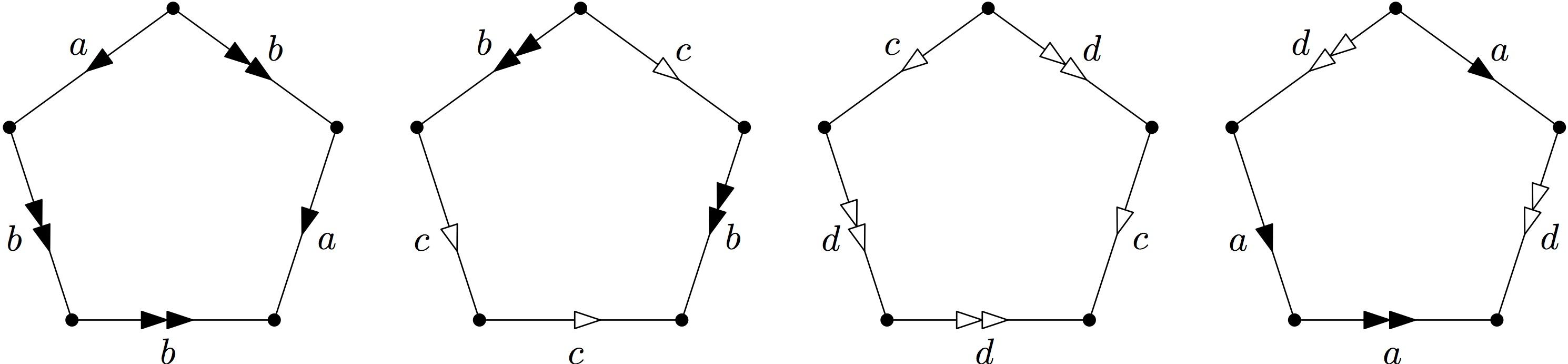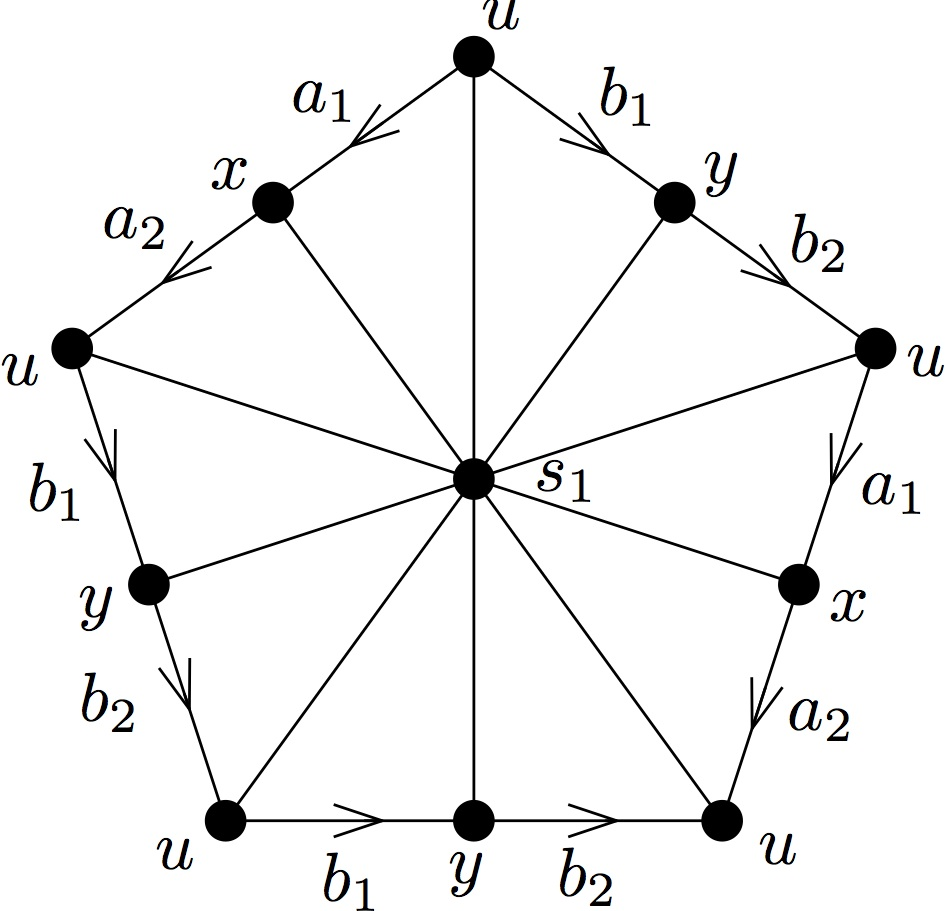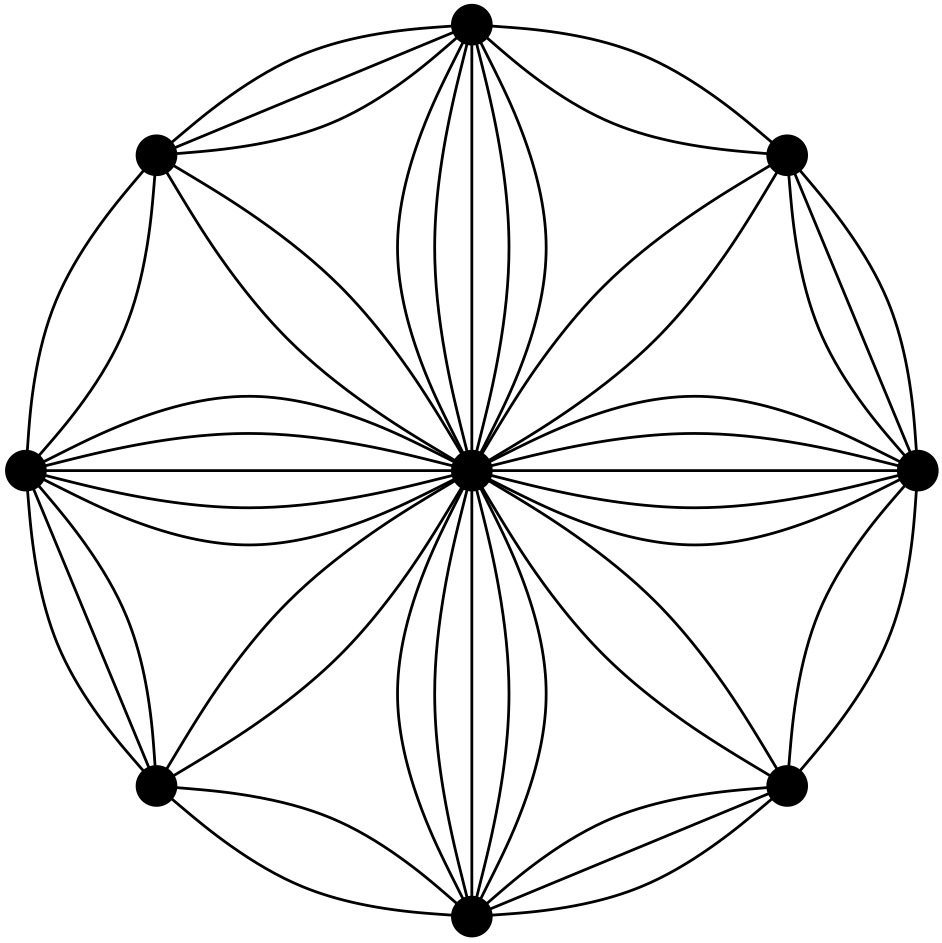# Which biased graphs are group-labelled graphs? A postscript: Do we really need infinite groups?

Guest post by Daryl Funk

This post is meant as a short postscript to Irene’s post in March, Which biased graphs are group-labelled graphs? Since Irene’s post generated a fair number of comments, there may be some interest in taking a closer look at the construction used in Theorem 1 of that post. Let us quickly remind ourselves of the context, and the theorem.

A graph $G$ together with a collection $\mathcal{B}$ of its cycles — called balanced — is a biased graph. Cycles not in $\mathcal{B}$ are unbalanced. Orienting the edges of $G$ and labelling each edge with an element of a group gives us a group-labelled graph. A group-labelled graph naturally gives rise to a biased graph, as follows. Traverse each cycle via a simple closed walk, taking the product of the group elements labelling each edge as you go, taking the inverse of the element when traversing an edge in reverse. Declare as balanced just those cycles for which such a product is the identity. Thus, group-labelled graphs provide the primary examples of biased graphs. An arbitrary biased graph $(G,\mathcal{B})$ is group-labellable if there is a group and a labelling for which this procedure yields precisely its collection of balanced cycles $\mathcal{B}$. Hence the question of our title: Which biased graphs are group-labelled? Theorem 1 provides an answer.

Theorem 1. Let $(G,\mathcal{B})$ be a biased graph. Construct a 2-cell complex $K$ from $G$ by adding a disc with boundary $C$ for each $C \in \mathcal{B}$. Then the following are equivalent:

1. $(G,\mathcal{B})$ is group-labellable.
2. $(G,\mathcal{B})$ is $\pi_1(K)$-labellable (where $\pi_1(K)$ is the fundamental group of $K$).
3. Every unbalanced cycle of $G$ is noncontractible in $K$.

Barring degenerate cases, the $\pi_1(K)$-labelling constructed by Theorem 1 is a labelling by an infinite group. In practice, we often work with graphs labelled by a finite group — probably the most studied group-labelled graphs are those labelled by the group of order two (i.e. signed graphs). Moreover, we consider only finite graphs. The following therefore is a natural question:

Question 1. If $(G,\mathcal{B})$ is group-labellable, is $(G,\mathcal{B})$ labellable by a finite group?

Could it be that there are group-labelled graphs whose collections of balanced cycles may only be realised by a labelling using an infinite group?

Given any group-labelling of a biased graph $(G,\mathcal{B})$, say using elements of the group $\Gamma$, there is a homomorphism from the fundamental group $\pi_1(K)$ of the 2-cell complex $K$ we construct in Theorem 1, to $\Gamma$. This homomorphism makes explicit the connection between the topology of $K$ and group-labellings of $(G,\mathcal{B})$. It also enables us to easily answer Question 1.

In preparation for a description of this homomorphism, let us recall some additional terminology and notation from Irene’s original post. Let $G$ be a graph and let $\Gamma$ be a group. Orient the edges of $G$, and let $\phi: E(G) \to \Gamma$ be a function. We extend $\phi$ to the set of closed walks in $G$ by defining, for closed walk $W$ with edge sequence $e_1, e_2, \ldots, e_k$,
$\phi(W) = \phi(e_1)^{\textrm{dir}(e_1)} \phi(e_2)^{\textrm{dir}(e_2)} \cdots \phi(e_k)^{\textrm{dir}(e_k)}$
where $\textrm{dir}(e_i)$ is 1 if $W$ traverses edge $e_i$ in the forward direction, and is $-1$ if $W$ traverses $e_i$ in the backward direction. Let $\mathcal{B}_\phi$ be the collection of cycles $C$ for which a simple closed walk $W$ traversing $C$ has $\phi(W)=1$. With this notation, $(G,\mathcal{B})$ is group-labellable if there is a group $\Gamma$ and labelling $\phi$ such that $\mathcal{B}_\phi = \mathcal{B}$. In this case we say the labelling $\phi$ realises $\mathcal{B}$, and that $(G,\mathcal{B})$ is $\Gamma$-labellable.

As Irene described near the end of her post, there is a fourth statement equivalent to statements 1, 2, and 3 of Theorem 1. To state it, we need just two more definitions. A rerouting of a closed walk $W$ is a closed walk $W’$ obtained from $W$ by replacing a subpath $P$ of $W$, say between vertices $u$ and $v$, with another $u$-$v$ path $P’$ that is internally disjoint from $P$. If $P \cup P’$ is a balanced cycle, then this is a balanced rerouting. Statement 4 below is equivalent to Statements 1, 2, and 3 of Theorem 1:

1. No unbalanced cycle can be moved to a balanced cycle via a sequence of balanced reroutings of closed walks.

We may now describe the homomorphism $\pi_1(K) \to \Gamma$, where $K$ is the 2-cell complex constructed from $(G,\mathcal{B})$ and $(G,\mathcal{B})$ is $\Gamma$-labellable. Let $\phi : E(G) \to \Gamma$ with $\mathcal{B}_\phi = \mathcal{B}$. The fundamental group $\pi_1(K)$ of $K$ is constructed in our proof of Theorem 1 with presentation in terms of generators $g_e$, one for each edge $e$ not in a chosen spanning tree of $G$, and relations among these generators given by simple closed walks around the cycles in $\mathcal{B}$. Each edge in the spanning tree is labelled by the group identity 1, and for each cycle in $\mathcal{B}$ we add a relation corresponding to the product of the generators labelling the edges as they are traversed by a simple closed walk around the cycle (as described by Irene in her original post; of course, details may be found in our paper [DFP14]). Let us denote this $\pi_1(K)$-labelling by $\rho : E(G) \to \pi_1(K)$. For an element $g \in \pi_1(K)$ expressed as the word $g = g_{e_1}^{a_1} g_{e_2}^{a_2} \cdots g_{e_m}^{a_m}$ for some generators $g_{e_1}, g_{e_2}, \ldots, g_{e_m}$ and integers $a_1, a_2, \ldots, a_m$, define $\eta : \pi_1(K) \to \Gamma$ by
$\eta(g) = \eta \left( g_{e_1}^{a_1} g_{e_2}^{a_2} \cdots g_{e_m}^{a_m} \right) = \phi \left( \rho^{-1}(g_{e_1})^{a_1} \rho^{-1}(g_{e_2})^{a_2} \cdots \rho^{-1}(g_{e_m})^{a_m} \right) .$

Theorem 2.The map $\eta:\pi_1(K) \to \Gamma$ is a group homomorphism.

Proof. Clearly $\eta$ is a homomorphism if it is well-defined. So suppose $g=$ $g_{n_1}^{a_{n_1}} g_{n_2}^{a_{n_2}} \cdots g_{n_m}^{a_{m_n}}$ $=$ $g_{k_1}^{a_{k_1}} g_{k_2}^{a_{k_2}} \cdots g_{k_p}^{a_{k_p}}$ are two words expressing the element $g \in \pi_1(K)$, where $g_{n_1}$, $g_{n_2}$, $\ldots$, $g_{n_m}$, $g_{k_1}$, $g_{k_2}$, $\ldots$, $g_{k_p}$ are generators and $a_{n_1}, a_{n_2}, \ldots$, $a_{n_m}, a_{k_1}, a_{k_2}, \ldots, a_{k_p}$ are integers. We show that
$\eta\left(g_{n_1}^{a_{n_1}} g_{n_2}^{a_{n_2}} \cdots g_{n_m}^{a_{m_n}} ( g_{k_1}^{a_{k_1}} g_{k_2}^{a_2} \cdots g_{k_p}^{a_{k_p}})^{-1}\right)=1,$
and so that $\eta$ maps both words $g_{n_1}^{a_{n_1}} g_{n_2}^{a_{n_2}} \cdots g_{n_m}^{a_{m_n}}$ and $g_{k_1}^{a_{k_1}} g_{k_2}^{a_2} \cdots g_{k_p}^{a_{k_p}}$ to the same element of $\Gamma$. We have
\begin{multline*}
\eta \left(g_{n_1}^{a_{n_1}} g_{n_2}^{a_{n_2}} \cdots g_{m_n}^{a_{m_n}} ( g_{k_1}^{a_{k_1}} g_{k_2}^{a_{k_2}} \cdots g_{k_p}^{a_{k_p}} )^{-1}\right) \\
= \phi \left( \rho^{-1}(g_{n_1})^{a_{n_1}} \rho^{-1}(g_{n_2})^{a_{n_2}} \cdots \rho^{-1}(g_{n_m})^{a_{n_m}} \rho^{-1}(g_{k_p})^{-a_{k_p}} \cdots \rho^{-1}(g_{k_2})^{-a_{k_2}} \rho^{-1}(g_{k_1})^{-a_{k_1}} \right) \\
= \phi\left( \rho^{-1}(g_{n_1})\right)^{a_{n_1}} \phi\left(\rho^{-1}(g_{n_2})\right)^{a_{n_2}} \cdots \phi\left(\rho^{-1}(g_{n_m})\right)^{a_{n_m}} \phi \left(\rho^{-1}(g_{k_p})\right)^{-a_{k_p}} \cdots \\ \phi\left(\rho^{-1}(g_{k_2})\right)^{-a_{k_2}} \phi\left(\rho^{-1}(g_{k_1})\right)^{-a_{k_1}}.
\end{multline*}
In $\pi_1(K)$, $g_{n_1}^{a_{n_1}} g_{n_2}^{a_{n_2}} \cdots g_{m_n}^{a_{m_n}} g_{k_p}^{-a_{k_p}} \cdots g_{k_2}^{-a_{k_2}} g_{k_1}^{-a_{k_1}} = 1$; the relations in $\pi_1(K)$ that reduce this word to the identity correspond to a sequence of reroutings via balanced cycles. The word in $\Gamma$ given by $\eta$ has the same corresponding edge sequence. Since $\mathcal{B}_\phi = \mathcal{B}$, this word is therefore reduced to the identity by the same sequence of balanced reroutings. $\square$

Let’s now use this homomorphism to answer Question 1.

Theorem 3. There exists a group-labellable biased graph whose collection of balanced cycles is not realised by any labelling with any finite group.

Proof. Let $H$ be the Higman group
$H = \left< a, b, c, d \mid a^{-1} b a = b^2, b^{-1} c b = c^2, c^{-1} d c = d^2, d^{-1} a d = a^2 \right>.$
The Higman group is infinite with no non-trivial finite quotients [H51] (the Wikipedia entry has a brief description). Construct a simplicial 2-complex $\mathcal{K}$ by identifying the points of edges marked $a$, $b$, $c$, and $d$, respectively, of five pentagons, oriented as shown in the figure.Let $G$ be the graph consisting of the vertices and edges of the barycentric subdivision of $\mathcal{K}$ (the following figure shows the part of the graph obtained from the first pentagon above), and let $\mathcal{B}$ be the set of cycles of $G$ that are contractible in $\mathcal{K}$.By construction, the fundamental group $\pi_1(\mathcal{K})$ is $H$. Hence the biased graph $(G,\mathcal{B})$ is $H$-labellable. Let $\Gamma$ be a group for which there is a labelling $\gamma : E(G) \to \Gamma$ realising $\mathcal{B}$. By Theorem 2, there is a homomorphism from $H$ to $\Gamma$. Since $H$ has no non-trivial finite quotient, and $(G,\mathcal{B})$ is not labellable by the trivial group, $\Gamma$ cannot be finite. $\square$

The graph $G$ constructed in the proof of Theorem 3 is pretty; it is shown below.# References

[H51] Graham Higman. A finitely generated infinite simple group. J. London Math. Soc., 26:61-64, 1951.

[DFP14] DeVos, Matthew; Funk, Daryl; and Pivotto, Irene. When does a biased graph come from a group labelling? Advances in Applied Mathematics, Vol 61 (October 2014), pp 1-18.

# Chromatic polynomials I

At the beginning of this year I started a new postdoc, still at the University of Western Australia and still with Gordon Royle, but on a new project, which is titled “Real chromatic roots of graphs and matroids”. That is, real roots of the chromatic polynomial of graphs and matroids. Since its first definition by Birkhoff in 1912, the chromatic polynomial has received much attention, not only by the mathematical community, but also, for example, by physicists. There are lots of interesting results and yet more interesting open problems in the area, and since I’m now navigating the extensive literature I decided to write a series of posts on the subject. I will mostly follow the notation of Gordon Royle’s excellent survey on chromatic and flow roots [R09].

Let’s start with a bit of history and some basic definitions. The chromatic polynomial (for planar graphs) was first defined by Birkhoff in an attempt to find an algebraic proof of the then open four-colour problem. The chromatic polynomial $P(G,q)$ of a graph $G$ counts, for every positive integer $q$, the number of proper colouring of the vertices of $G$ with $q$ colours. One can easily check that, if $e$ is an edge of $G$, then $P(G, q)=P(G\backslash e, q)-P(G/e, q).$ In fact, the number of $q$-colouring of $G$ is equal to the number of $q$-colouring of $G\backslash e$ where the endpoints of $e$ receive distinct colours and $P(G/e,q)$ counts the number of $q$-colourings of $G \backslash e$ where the endpoints of $e$ receive the same colour. The above formula is called the deletion-contraction formula and from it one can easily deduce that $P(G,q)$ is indeed a polynomial. One can also see that this is the case by showing directly that $P(G,q)$ is a sum of polynomials, as in the next lemma.

Lemma 1: For every graph $G$, $P(G,q)=\sum_{X\subseteq E(G)}(-1)^{|X|}q^{k(X)},$ where $k(X)$ is the number of components of the graph induced by $X$.

proof: Let us consider colourings (not necessarily proper ones) of $V(G)$ with $q$ colours; there are $q^{|V(G)|}$ of them. For every $e \in E(G)$, denote by $B_e$ the set of colourings where the endpoints of $e$ receive the same colour. Then $P(G,q)=q^{|V(G)|}-|\cup_{e\in E(G)}B_e|.$ Also note that $|\cap_{e\in X}B_e|=q^{k(X)}.$ Now the result follows from applying the inclusion-exclusion principle to the first equality and using the second equality.$\qquad \Box$

Since the chromatic polynomial can be evaluated at real and complex values, Birkhoff hoped to use analytic methods to prove the 4-colour conjecture. In fact, the four-colour Theorem is equivalent to stating that $P(G,4)>0$ for all planar graphs $G$, i.e. 4 is not a chromatic root of any planar graph. As we know, such an analytic proof was never found, but Birkhoff and Lewis [BL46] did show that if $G$ is planar then $P(G,q)>0$ for all real $q\geq 5$. They conjectured that 5 could be changed to 4, and almost 70 years later their conjecture is still open.

Conjecture 2 (Birkhoff-Lewis, 1946). If $G$ is a planar graph, then $P(G,q)>0$ for all real $q \geq 4$.

The Birkhoff-Lewis conjecture motivated the study of real chromatic roots of graphs, with special attention to finding lower and upper bounds on them. A real interval $(a,b)$ is a root-free interval for a family of graphs $\mathcal{G}$ if no graph in $\mathcal{G}$ has a chromatic root (i.e. a root of its chromatic polynomial) in the interval $(a,b)$.

The chromatic polynomial of a graph $G$ with $n$ vertices may be expressed as $P(G,q)=\sum_{i=1}^n (-1)^{n-i}h_iq^i,$ where $h_i \in \mathbb{N}$ for all $i=1,\ldots,n$ and $h_n=1$. From this one can easily deduce that $(-1)^nP(G,q)>0$ for all real $q<0$, thus the interval $(-\infty,0)$ is a root-free interval for all graphs. With a bit more work (considering forests first, then using induction and the deletion-contraction formula) one can also show that $(-1)^{n-k(G)}P(G,q)>0$ for all real $q \in (0,1)$. A much less trivial result, due to Jackson [J93] gives the following surprising root-free interval.

Theorem 3 (Jackson [J93]). Let $G$ be a connected graph with $n\geq 2$ vertices and $b$ blocks. Then $(-1)^{n+b+1}P(G,q)>0$ for all real $q \in (1,32/27]$.

What is especially surprising about this result is the fact that this interval is best possible, in  the following sense.

Theorem 4 (Thomassen, [T97]). For all real numbers $a,b$ with $32/27<a<b$, there exists a graph $G$ that has a chromatic root in $[a,b]$.

What about largest real roots? A family of graphs $\mathcal{G}$ has an upper root-free interval if there is a real number $a$ such that no graph in $\mathcal{G}$ has a real root larger than $a$. The class of all graphs does not have an upper root-free interval (since you can get arbitrarily large chromatic roots from cliques). Restricting our attention to bipartite graphs, the situation does not improve.

Theorem 5 (Woodall [W77]). If $n$ is sufficiently large compared to $m$, then the complete bipartite graph $K_{m,n}$ has real chromatic roots arbitrarily close to all integers in $[2,m/2]$.

However, the situation is quite different when we consider proper minor-closed classes of graphs instead. This is because graphs in a proper minor-closed class must have a vertex of “small” degree.

Theorem 6 (Mader [M67]). For every $k \in \mathbb{N}$ there exists an integer $f(k)$ such that any graph with minimum degree at least $f(k)$ has a $K_k$-minor.

It follows that, for every proper minor-closed family of graphs $\mathcal{G}$, there exists a smallest integer $d=d(\mathcal{G})$ such that every graph in $\mathcal{G}$ has a vertex of degree at most $d$. Woodall and Thomassen proved the next result independently.

Theorem 7 (Thomassen [T97], Woodall [W97]). If $\mathcal{G}$ is a proper minor-closed family of graphs, then $(d(\mathcal{G}),\infty)$ is an upper root-free interval for $\mathcal{G}$.

In the next post I’ll talk about complex chromatic roots and then move on to matroids.

# References:

[BL46] G.D. Birkhoff, D.C. Lewis, Chromatic polynomials. Trans. Amer. Math. Soc. 60, (1946). 355–451.

[J93] B. Jackson, A zero-free interval for chromatic polynomials of graphs. Combin. Probab. Comput. (1993), no. 3, 325–336.

[R09] G.F. Royle, Recent results on chromatic and flow roots of graphs and matroids. Surveys in combinatorics 2009, 289–327, London Math. Soc. Lecture Note Ser., 365, Cambridge Univ. Press, Cambridge, 2009.

[T97] Thomassen, Carsten, The zero-free intervals for chromatic polynomials of graphsCombin. Probab. Comput. 6 (1997), no. 4, 497–506.

[W77] D.R. Woodall, Zeros of chromatic polynomials. Combinatorial surveys (Proc. Sixth British Combinatorial Conf., Royal Holloway College, Egham, 1977), pp. 199–223. Academic Press, London, 1977.

[W97] D.R. Woodall, The largest real zero of the chromatic polynomial. Discrete Math. 172 (1997), no. 1-3, 141–153.